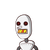# write the polynomial p(x)=2x^2 -7x-15as a product of two first degree polynomial? plz replay guyzz ​

write the polynomial p(x)=2x^2 -7x-15as a product of two first degree polynomial?
plz replay guyzz ​

### 1 thought on “write the polynomial p(x)=2x^2 -7x-15as a product of two first degree polynomial?<br /> plz replay guyzz ​”

1.